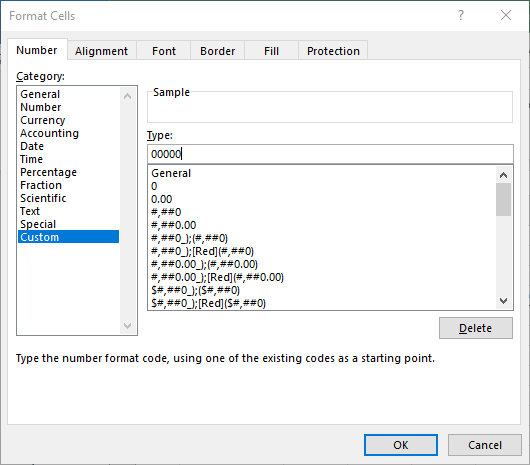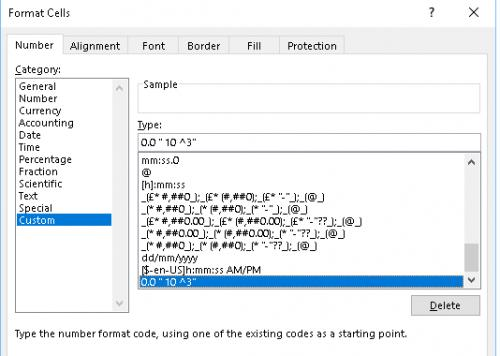Please Note: This article is written for users of the following Microsoft Excel versions: 2007, 2010, 2013, 2016, 2019, and Excel in Office 365. If you are using an earlier version (Excel 2003 or earlier), this tip may not work for you. For a version of this tip written specifically for earlier versions of Excel, click here: Engineering Calculations.

# Engineering Calculationsby Allen Wyatt
(last updated June 29, 2019)

In an engineering environment, it is not unusual to need to "normalize" numbers in some manner. For instance, you may need to show numeric values normalized to multiples of 10^3, such that 7340 is expressed as 7.34 and 73400 is expressed as 73.4.

It is possible in Excel to use a custom number format to express information in scientific notation that will normalize the display of a number to a multiple of 10^3. To do this, you would follow these steps:

1. Select the cells you want formatted.
2. Display the Home tab of the ribbon.
3. Click the small icon at the lower-right corner of the Number group. Excel displays the Format Cells dialog box.
4. Make sure the Number tab is selected. (See Figure 1.)
5.Figure 1. The Number tab of the Format Cells dialog box.

6. In the list of format categories, choose Custom.
7. In the Type box, enter ##0.0E+0 as your format. (This provides only one number to the right of the decimal place. If you want more, increase the number of zeros after the decimal place.)
8. Click on OK.

Now, when you enter a number such as 7340 into the cell, Excel displays it as 7.3E+3. Because of the way the cell format was entered, the portion after the E will always be a multiple of 3.

This is fine and good, but what if you want just the 7.3 in the cell, and then a metric prefix with a unit in an adjoining cell, such as kilograms? This is a bit more complex, but it can be done using formulas. For instance, let's assume you have your original number in cell A2, you wanted the normalized number in cell B2, and the metric prefix and unit name in cell C2. All you would need to do is enter the following formula in cell B2:

```=IF(OR(A2>=1,A2<=-1),SIGN(A2)*(ABS(A2)/(10^(3*INT(LOG(ABS(A2))/3))))),
IF(A2=0,0,SIGN(A2)*(ABS(A2)*10^(-3*INT(LOG(ABS(A2))/3)))))
```

Assuming the units you are working with are an imaginary unit called a foo, in cell C2 you would use a different formula, as follows:

```=IF(OR(A2>=1, A2<=-1),CHOOSE(INT(LOG(ABS(A2))/3)+1, "Foos", "Kilofoos",
"Megafoos", "Gigafoos", "Terafoos", "Petafoos", "Exafoos"),
IF(A2=0,"",CHOOSE(INT(-LOG(ABS(A2))/3)+1, "Millifoos", "Microfoos",
"Nanofoos", "Picofoos", "Femtofoos", "Attofoos")))
```

These formulas may seem a bit long, and they are. However, they will work for any number between approximately -9.99999E-18 to 9.99999E+20. For instance, if you put the number .000125 in cell A2, then cell B2 will contain 125 and cell C2 would contain Millifoos.

If you prefer to not use longer formulas such as these in your workbooks, you can develop a couple of VBA functions to do the trick. The following function, MySciNum, returns a normalized number. Thus, you would use =MySciNum(A2) in cell B2 to get the same results as noted above:

```Function MySciNum(BaseNum As Double) As Double
Select Case BaseNum
Case Is >= 1
While Abs(BaseNum) > 1000
BaseNum = BaseNum / 1000
Wend
Case 0
'Do nothing
Case Else
While Abs(BaseNum) < 1
BaseNum = BaseNum * 1000
Wend
End Select
MySciNum = BaseNum
End Function
```

This function only returns a number. To return the units with the appropriate metric prefix, you would use the following function. All you need to do is pass it the cell reference and the name of a single unit. For instance, you could use =MySciPre(A2, "foo"). The macro is as follows:

```Function MySciPre(BaseNum As Double, Unit As String) As String
Dim OrigNum As Double
Dim Pref As Integer
Dim Temp As String

Pref = 0
OrigNum = BaseNum
Select Case BaseNum
Case Is >= 1
While Abs(BaseNum) > 1000
BaseNum = BaseNum / 1000
Pref = Pref + 1
Wend
Case 0
Pref = 99
Case Else
While Abs(BaseNum) < 1
BaseNum = BaseNum * 1000
Pref = Pref - 1
Wend
End Select

Select Case Pref
Case -6
Temp = "atto" & Unit
Case -5
Temp = "femto" & Unit
Case -4
Temp = "pico" & Unit
Case -3
Temp = "nano" & Unit
Case -2
Temp = "micro" & Unit
Case -1
Temp = "milli" & Unit
Case 0
Temp = Unit
Case 1
Temp = "kilo" & Unit
Case 2
Temp = "mega" & Unit
Case 3
Temp = "giga" & Unit
Case 4
Temp = "tera" & Unit
Case 5
Temp = "peta" & Unit
Case 6
Temp = "exa" & Unit
Case Else
Temp = ""
End Select

If Len(Temp) > 0 Then
Temp = LCase(Temp)
Temp = UCase(Left(Temp, 1)) & Mid(Temp, 2)
If Abs(OrigNum) <> 1 Then Temp = Temp & "s"
End If

MySciPre = Temp
End Function
```

Note:

If you would like to know how to use the macros described on this page (or on any other page on the ExcelTips sites), I've prepared a special page that includes helpful information. Click here to open that special page in a new browser tab.

ExcelTips is your source for cost-effective Microsoft Excel training. This tip (12874) applies to Microsoft Excel 2007, 2010, 2013, 2016, 2019, and Excel in Office 365. You can find a version of this tip for the older menu interface of Excel here: Engineering Calculations.

##### Author Bio

Allen Wyatt

With more than 50 non-fiction books and numerous magazine articles to his credit, Allen Wyatt is an internationally recognized author. He is president of Sharon Parq Associates, a computer and publishing services company. ...

##### MORE FROM ALLEN

Making Multiple Worksheet Copies

If you spend a lot of time creating a worksheet, you might want to make multiple copies of that worksheet as a starting ...

Discover More

Need to add a hyperlink to a comment? It's easy to do by following the steps outlined in this tip.

Discover More

Working with Multiple Printers

If you have multiple printers accessible to your computer, you may need a way to quickly print your worksheet on a ...

Discover MoreProfessional Development Guidance! Four world-class developers offer start-to-finish guidance for building powerful, robust, and secure applications with Excel. The authors show how to consistently make the right design decisions and make the most of Excel's powerful features. Check out Professional Excel Development today!

##### More ExcelTips (ribbon)

Finding the Path to the Desktop

Figuring out where Windows places certain items (such as the user's desktop) can be a bit frustrating. Fortunately, there ...

Discover More

Stepping Through a Non-Contiguous Range of Cells

Using macros to step through each cell in a selection is a common occurrence. What if that selected range is made up of ...

Discover More

Saving an Unsavable Workbook

Macros can allow you to do some fancy data validation in your workbooks, such as checking to see if the user entered ...

Discover More
##### Subscribe

FREE SERVICE: Get tips like this every week in ExcelTips, a free productivity newsletter. Enter your address and click "Subscribe."

If you would like to add an image to your comment (not an avatar, but an image to help in making the point of your comment), include the characters [{fig}] in your comment text. You’ll be prompted to upload your image when you submit the comment. Maximum image size is 6Mpixels. Images larger than 600px wide or 1000px tall will be reduced. Up to three images may be included in a comment. All images are subject to review. Commenting privileges may be curtailed if inappropriate images are posted.

What is two less than 4?

2019-07-29 12:08:17

Peter Atherton

Lars
When it is that important we can alway try a macro or UDF. We can't apply formatting with a function but, if you had a lot of numbers, we could write a procedure to change the format later.

Function N23Sig(ByVal ref As Range) As Double
Dim tmp As Double, L As Integer
If Len(ref) < 4 Then
N23Sig = Application.Round(ref / 1000, 2)
Exit Function
End If
tmp = ref / 1000
L = Len(tmp)
If L > 4 Then
N23Sig = Left(tmp, 4)
Else
N23Sig = tmp
End If
End Function

PS. You could write to Microsoft suggesting this option.
HTH

PPS. Actually, this formula works but I got it after writing the function. Moral; keep away from TEXT functions!
=LEFT(ROUND(I18/1000,2),4)*1

2019-07-29 01:56:37

Lars

Peter.

I've done something similar but, as you write, it's no longer numeric.

I cannot understand why Excel does not have the option "number significant digits".

2019-07-25 11:30:52

Peter Atherton

Lars
We can use the TEXT function to get what you want but if will convert is to text and you will lose the number. See if you can live with the following:
=IF(LEN(G2)=4,TEXT(ROUND(G2/1000,2),"0.00 10^3"),(ROUND(G2/1000,(LEN(G2)-4))))

[{Fig}]

2019-07-25 11:29:38

Peter Atherton

Lars
We can use the TEXT function to get what you want but if will convert is to text and you will lose the number. See if you can live with the following:
=IF(LEN(G2)=4,TEXT(ROUND(G2/1000,2),"0.00 10^3"),(ROUND(G2/1000,(LEN(G2)-4))))

[{Fig}]

2019-07-25 03:53:57

Lars

Peter.

Nice solution of 10^3.

My real question was how to get 3 significant digits without having to change number of decimals.

2019-07-24 12:05:59

Peter Atherton

Lars
Try this formula, with n in B2:
=IF(LEN(B2)=4,B2/1000,(ROUND(B2/1000,(LEN(B2)-4))))

And use this custom Format as shown:

(see Figure 1 below)

You will need to increase the decimals for the second numberFigure 1. Custom Format "10^3"

2019-07-24 09:32:15

MIchael Armstrong

Beautiful; would have take me days to do that, if I hadn't given up altogether. Not looking forward to the day I have to deal with Exafoos.

2019-07-23 06:45:35

Lars

How do I accomplish "7340 is expressed as 7.34 10^3 and 73400 is expressed as 73.4 10^3", that is allways 3 significant digits, without using VBA.

##### This Site

Got a version of Excel that uses the ribbon interface (Excel 2007 or later)? This site is for you! If you use an earlier version of Excel, visit our ExcelTips site focusing on the menu interface.